Open In App

# Top Data Structures That Every Programmer Must Know

A Data Structure organizes and stores data in a computer so that we can perform operations on the data more efficiently. There are many diverse applications of data structures in Computer Science and Software Engineering. The use of data structures is most common in all computer programs and software systems. As well, data structures are an important part of the fundamentals of Computer Science and Software Engineering. There is no doubt that this topic is a key component of Software Engineering and also very important from the perspective of interview preparation. Therefore we must have good knowledge about data structure, In this post, we are going to discuss with you the top Data structure that every programmer must know. The knowledge of top data structure also becomes important for the implementation of the advanced data structure.Top Data structure that every programmer must know

## What is data structure?

A data structure is the mathematical or logical model of an organization of data. In short, a data structure is a way to organize data in a form that is accessible to computers. It allows the processing of a large amount of data in a relatively short period of time. The main purpose of using data structures is to reduce time and space complexities. An efficient data structure makes use of minimum memory space and takes the minimal possible time to execute.

## Top Data structure that every programmer must know

Now, as we know about data structure and its importance, let’s take a look at the most common Data structure that every programmer must know:

## 1. Array

An array is a collection of items of the same variable type stored that are stored at contiguous memory locations. It’s one of the most popular and simple data structures and is often used to implement other data structures. Each item in an array is indexed starting with 0.

### Why Array Data Structures is needed?

Assume there is a class of five students and if we have to keep records of their marks in examination then, we can do this by declaring five variables individual and keeping track of records but what if the number of students becomes very large, it would be challenging to manipulate and maintain the data.

### Types of arrays:

There are majorly two types of arrays: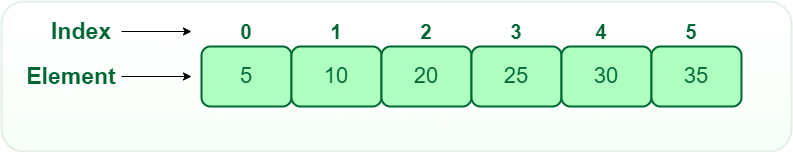One Dimensional Array

• Two-dimensional array: 2-D Multidimensional arrays can be considered as an array of arrays or as a matrix consisting of rows and columns.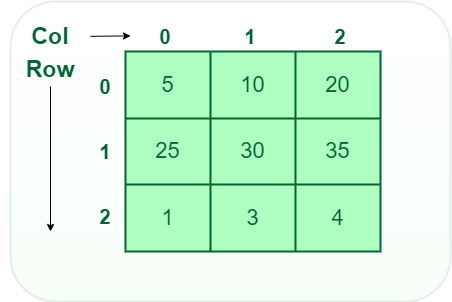Two-Dimensional Array

• Three-dimensional array: A 3-D Multidimensional array contains three dimensions, so it can be considered an array of two-dimensional arrays.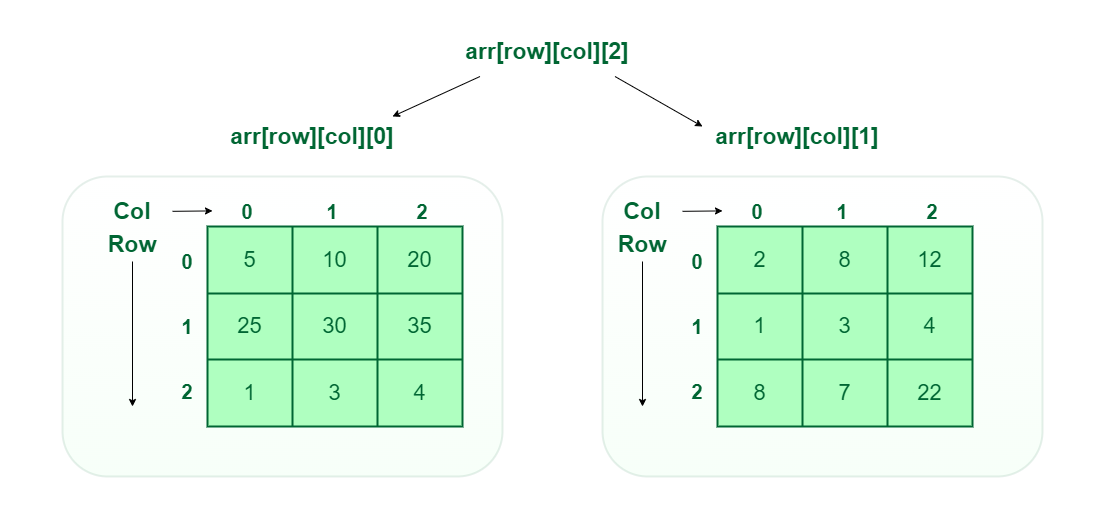Three-Dimensional Array

### Types of Array operations:

• Traversal: Traverse through the elements of an array.
• Insertion: Inserting a new element in an array.
• Deletion: Deleting element from the array.
• Searching:  Search for an element in the array.
• Sorting: Maintaining the order of elements in the array.

• Arrays allow random access to elements. This makes accessing elements by position faster.
• Arrays have better cache locality which makes a pretty big difference in performance.
• Arrays represent multiple data items of the same type using a single name.

### Application of array:

• They are used in the implementation of other data structures such as array lists, heaps, hash tables, vectors, and matrices.
• Database records are usually implemented as arrays.
• It is used in lookup tables by computer.
• It is used for different sorting algorithms such as bubble sort insertion sort, merge sort, and quick sort.

## 2. String

Strings are defined as an array of characters. The difference between a character array and a string is the string is terminated with a special character ‘\0’.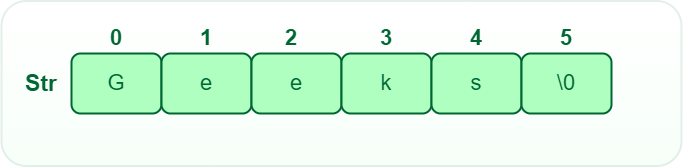String data structure

Declaring a string is as simple as declaring a one-dimensional array. Below is the basic syntax for declaring a string in C programming language.

`char str_name[size];`

### String operations:

• Substrings:  A substring is a contiguous sequence of characters within a string
• Concatenation: This operation is used for appending one string to the end of another string.
• Length: It defines the number of characters in the given string.
• Text Processing Operations: Text processing is the process of creating and editing strings.
• Insertion: This operation is used to insert characters in the string at the specified position.
• Deletion: This operation is used to delete characters in the string at the specified position.
• Update: This operation is used to update characters in the string at the specified position.

• String provides us with a string library to create string objects which will allow strings to be dynamically allocated and also boundary issues are handled inside the class library.
• String provides us various inbuilt functions under string library such as sort(), substr(i, j), compare(), push_back() and many more.
• The string helps as a base for many data structures such as tries, suffix trees, suffix arrays, ternary search trees, and much more.
• Strings provide us with very helpful string algorithms for solving very complex problems with less time complexity.

### Applications of String:

• Plagiarism Checker: Strings can be used to find Plagiarism in codes, and contents in a very little amount of time using string matching algorithms. Using this the computer can easily tell us the percentage of the code, and what percentage matches the text typed by any two users.
• Encoding/Decoding(Cipher Text Generation): Strings can be used for encoding and decoding for the safe transfer of data from sender to receiver to make sure no one in the way of transmission gets to read your data as they could perform both active and passive attacks. The text you transfer as a message gets ciphered at the sender’s end and decoded at the receiver’s end.
• Information Retrieval: String applications help us to retrieve information from unknown data sources( large datasets used as input) along with the help of a string matching/retrieval module helps us to retrieve important information.
Improved Filters For The Approximate Suffix-Prefix Overlap Problem: Strings and its algorithms applications help us to provide improved Filters for the Approximate Suffix-Prefix Overlap Problem.

### Most Commonly asked interview questions on String:

A linked list is a linear data structure, Unlike arrays, linked list elements are not stored at a contiguous location. it is basically chains of nodes, each node contains information such as data and a pointer to the next node in the chain. In the linked list there is a head pointer, which points to the first element of the linked list, and if the list is empty then it simply points to null or nothing.

### Why to linked list data structure needed?

Here are a few advantages of a linked list that is listed below, it will help you understand why it is necessary to know.

• Dynamic Data structure: The size of memory can be allocated or de-allocated at run time based on the operation insertion or deletion.
• Ease of Insertion/Deletion: The insertion and deletion of elements are simpler than arrays since no elements need to be shifted after insertion and deletion, Just the address needed to be updated.
• Efficient Memory Utilization: As we know Linked List is a dynamic data structure the size increases or decreases as per the requirement so this avoids the wastage of memory.
• Implementation: Various advanced data structures can be implemented using a linked list like stack, queue, graph, hash maps, etc.

There are mainly three types of linked lists:

• Singly-linked list: Traversal of items can be done in the forward direction only due to the linking of every node to its next node.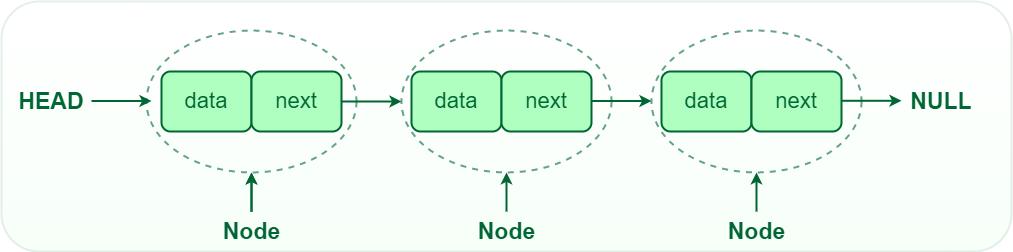• Doubly linked list: Traversal of items can be done in both forward and backward directions as every node contains an additional prev pointer that points to the previous node.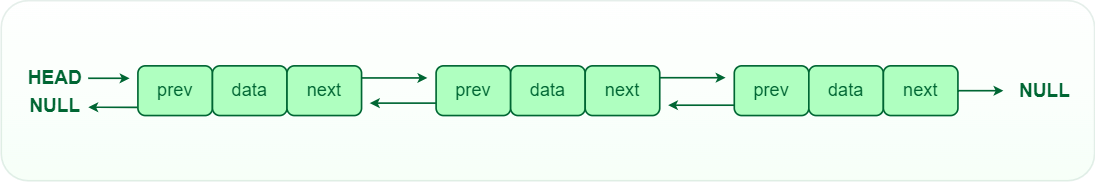• Circular linked lists: A circular linked list is a type of linked list in which the first and the last nodes are also connected to each other to form a circle, there is no NULL at the end.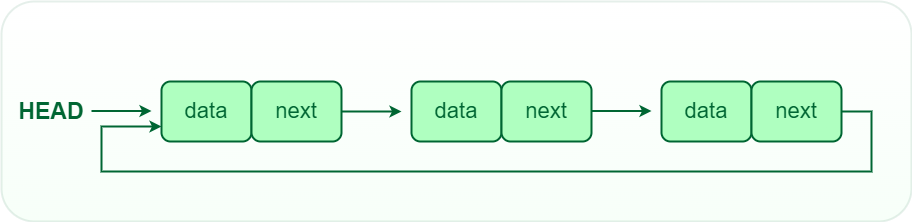• Traversal: We can traverse the entire linked list starting from the head node. If there are n nodes then the time complexity for traversal becomes O(n) as we hop through each and every node.
• Insertion: Insert a key to the linked list. An insertion can be done in 3 different ways; insert at the beginning of the list, insert at the end of the list and insert in the middle of the list.
• Deletion: Removes an element x from a given linked list. You cannot delete a node by a single step. A deletion can be done in 3 different ways; delete from the beginning of the list, delete from the end of the list and delete from the middle of the list.
• Search: Find the first element with the key k in the given linked list by a simple linear search and returns a pointer to this element

• Insertion and deletion in linked lists are very efficient.
• Linked lists are used for dynamic memory allocation which means effective memory utilization hence, no memory wastage.
• For the implementation of stacks and queues and for the representation of trees and graphs.
• The linked list can be expanded in constant time.

Here are some of the applications of a linked list:

• Linear data structures such as stack, queue, and non-linear data structures such as hash maps, and graphs can be implemented using linked lists.
• Dynamic memory allocation: We use a linked list of free blocks.
• Implementation of graphs: Adjacency list representation of graphs is the most popular in that it uses linked lists to store adjacent vertices.
• In web browsers and editors, doubly linked lists can be used to build a forward and backward navigation button.
• A circular doubly linked list can also be used for implementing data structures like Fibonacci heaps.

## 4. Stack

Stack is a linear data structure in which insertion and deletion are done at one end this end is generally called the top. It works on the principle of Last In First Out (LIFO) or First in Last out (FILO). LIFO means the last element inserted inside the stack is removed first. FILO means, the last inserted element is available first and is the first one to be deleted.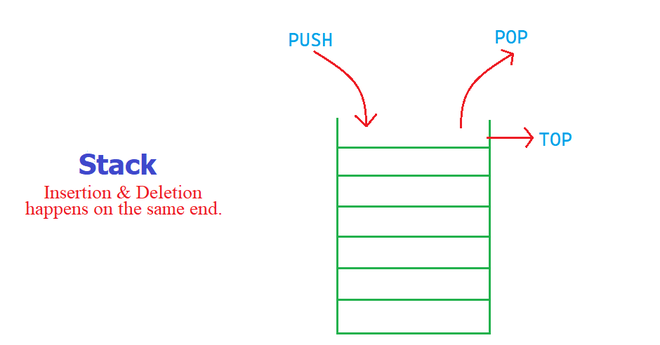Stack Data Structure

### Operations in a Stack:

1. Push: Add an element to the top of a stack
2. Pop: Remove an element from the top of a stack
3. IsEmpty: Check if the stack is empty
4. IsFull: Check if the stack is full
5. top/Peek: Get the value of the top element without removing it

• Stack helps in managing data that follows the LIFO technique.
• Stacks are be used for systematic Memory Management.
• Stacks are more secure and reliable as they do not get corrupted easily.
• Stack allows control over memory allocation and deallocation.
• Stack cleans up the objects automatically.

### Applications of Stack Data Structure:

• Stack is used for evaluating expression with operands and operations.
• Matching tags in HTML and XML
• Undo function in any text editor.
• Compilers use the stack to calculate the value of expressions like 3 + 4 / 7 * (2 – 1) by converting the expression to prefix or postfix form.
• Stacks help in reversing any set of data or strings.

## 5. Queue

A Queue is a linear structure that follows a particular order in which the operations are performed. The order is First In First Out (FIFO). It is similar to the ticket queue outside a cinema hall, where the first person entering the queue is the first person who gets the ticket.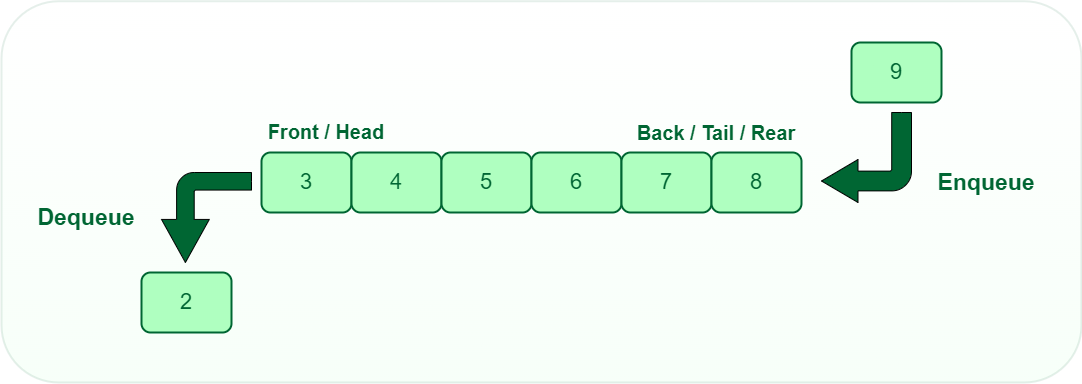Queue Data structure

### Operations of Queue:

A queue is an object (an abstract data structure – ADT) that allows the following operations:

1. Enqueue: Add an element to the end of the queue
2. Dequeue: Remove an element from the front of the queue
3. IsEmpty: Check if the queue is empty
4. IsFull: Check if the queue is full
5. top/Peek: Get the value of the front of the queue without removing it

### Types of queues:

• Simple Queue: In a simple queue, insertion takes place at the rear and removal occurs at the front. It strictly follows the FIFO (First in First out) rule.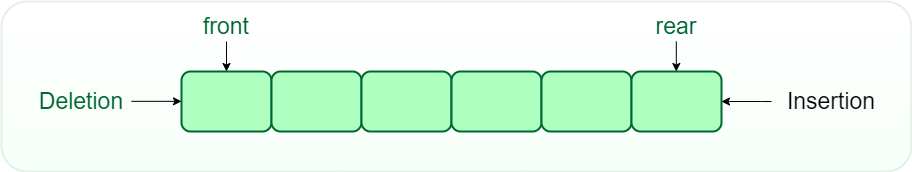Simple Queue

• Circular Queue: In a circular queue, the last element points to the first element making a circular link.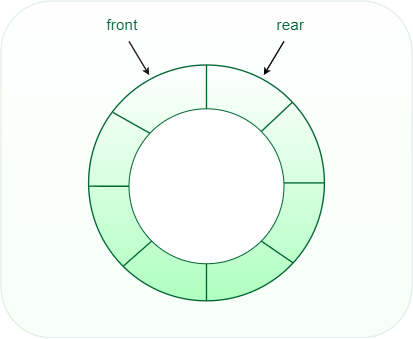Circular Queue

• Priority Queue: In a priority queue, the nodes will have some predefined priority in the priority queue. The node with the least priority will be the first to be removed from the queue. Insertion takes place in the order of arrival of the nodes.
• Double-Ended Queue: In a double-ended queue, insertion and removal of elements can be performed from either the front or rear. So, we can say that it does not follow the FIFO (First In First Out) rule.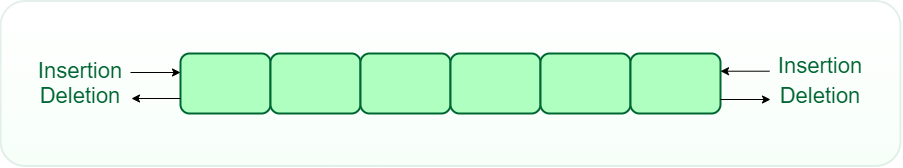Double-Ended Queue

• A large amount of data can be managed efficiently with ease.
• Operations such as insertion and deletion can be performed with ease as it follows the first in first out rule.
• Queues can be used in the implementation of other data structures.
• Queues are useful when a particular service is used by multiple consumers.
• Queues are fast in speed for data inter-process communication.

### Applications of Queue:

• CPU scheduling, Disk Scheduling
• When data is transferred asynchronously between two processes. The queue is used for synchronization. For example IO Buffers, pipes, file IO, etc
• Handling of interrupts in real-time systems.
• Call Center phone systems use Queues to hold people calling them in order.

## 6. Tree

A tree is non-linear and a hierarchical data structure consisting of a collection of nodes such that each node of the tree stores a value and a list of references to other nodes (the “children”).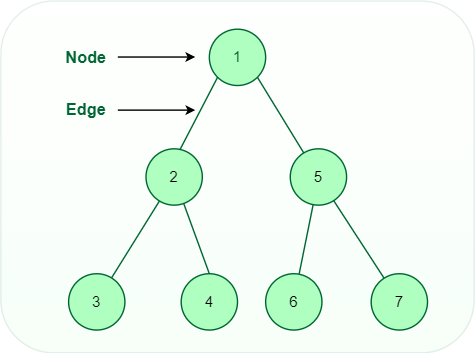Tree data structure

### Operations on tree data structure:

• Insert: Inserts an element in a tree/create a tree.
• Search: Searches an element in a tree.
• Tree Traversal: The tree traversal algorithm is used in order to visit a specific node in the tree to perform a specific operation on it.

### Advantage of tree data structure:

• Trees provide a hierarchical representation of the data.
• Trees are dynamic in nature so the number of nodes is not limited.
• Insertion and deletion in a tree can be done in moderate time.

### Applications of Tree data structure:

• Trees can be used to store data which are in hierarchical form.
• Different types of trees are used in various fields like databases, computer graphics, and computer networks.
• Tree data structures are used by operating systems to manage the file directories.

## 7. Heap

A Heap is a special Tree-based data structure in which the tree is a complete binary tree.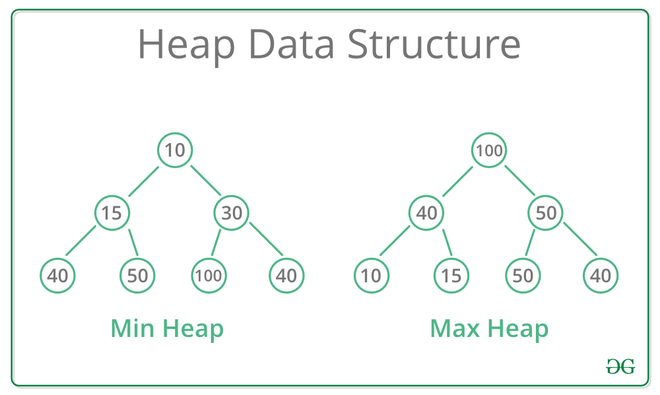Heap Data Structure

### Types of Heap Data Structure:

• Max-Heap: In a Max-Heap the key present at the root node must be the greatest among the keys present at all of it’s children. The same property must be recursively true for all sub-trees in that Binary Tree.
• Min-Heap: In a Min-Heap the key present at the root node must be minimum among the keys present at all of it’s children. The same property must be recursively true for all sub-trees in that Binary Tree.

### Operation on heap data structure:

• Heapify: a process of creating a heap from an array.
Insertion: process to insert an element in existing heap time complexity O(log N).
• Deletion: deleting the top element of the heap or the highest priority element, and then organizing the heap and returning the element with time complexity O(log N).
• Peek: to check or find the most prior element in the heap, (max or min element for max and min heap).

### Advantages of Heap Data Structure:

• It maintains the element according to their priority.
• The time complexity to just peek at the most prior element is constant O(1).
• It takes less time complexity, for inserting or deleting an element in the heap the time complexity is just O(log N).
• A binary heap is a balanced binary tree, and it is easy to implement.
• Heap data structure efficiently use graph algorithm such as Dijkstra.

### Application of Heap Data Structure:

• Heap is used to construct a priority queue.
• Heap sort is one of the fastest sorting algorithms with time complexity of O(N* log(N), and it’s easy to implement.
• Best First Search (BFS) is an informed search, where unlike the queue in Breadth-First Search, this technique is implemented using a priority queue.

## 8. Graph

A Graph is a non-linear data structure consisting of nodes and edges. The nodes are sometimes also referred to as vertices and the edges are lines or arcs that connect any two nodes in the graph. More formally a Graph can be defined as, A Graph consisting of a finite set of vertices(or nodes) and a set of edges that connect a pair of nodes.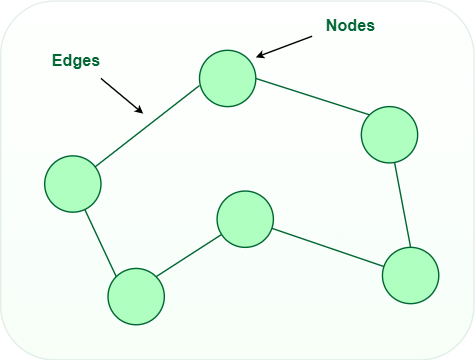Graph Data structure

### Graph Representation

In the graph data structure, a graph representation is a technique to store graphs in the memory of the computer. There are many ways to represent a graph:

The following two are the most commonly used representations of a graph.

1. Adjacency Matrix: An adjacency matrix represents a graph as a matrix of boolean values (0s and 1s). In a computer, a finite graph can be represented as a square matrix, where the boolean value indicates if two vertices are connected directly.
2. Adjacency List: An adjacency list represents a graph as an array of linked lists where an index of the array represents a vertex and each element in its linked list represents the other vertices that are connected with the edges, or say its neighbor.

### Types of Graphs

Based on the direction of edges, there are two types of graphs:

• Undirected Graph: A graph in which all the edges are bi-directional and the edges are not directed in any specific direction to vertices.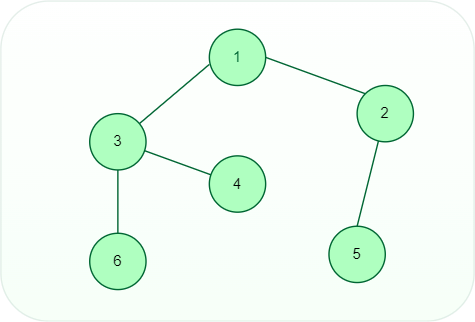Undirected graph

• Directed Graph: A graph in which all the edges are uni-directional and the edges are directed to some specific vertex.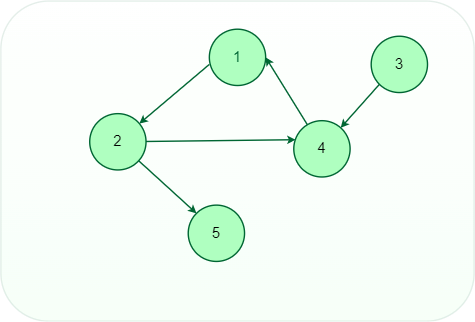Directed graph

Based on the weight of edges, there are two types of graphs:

• Weighted Graph:  A graph in which every edge has a value or weight and the values can represent quantities such as cost, distance, and time.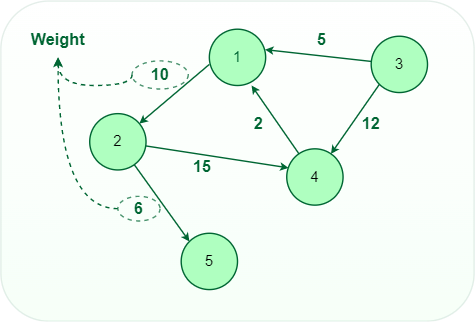Weighted graph

• Unweighted Graph: A graph in which there is no value or weight associated with the edge. All the graphs are said to be unweighted by default unless there is a value associated.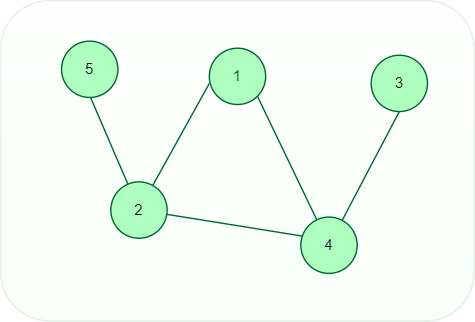Unweighted graph

### Graph Operations:

• Check if the graph contains a given value.
• Find the path from one vertex to another vertex.

• By using graphs we can easily find the shortest path, neighbours of the nodes, and many more.
• Graphs are used to implement algorithms like DFS and BFS.
• It helps in organizing data.
• It is used to find a minimum spanning tree which has many practical applications.
• Because of its non-linear structure, helps in understanding complex problems and their visualization.

### Applications of Graphs:

• Graphs are used to represent networks of communication.
• Graph theory is used to find the shortest path in a road or a network.
• Graphs are used to represent networks of communication
• In Google Maps, various locations are represented as vertices or nodes and the roads are represented as edges graph theory is used to find the shortest path between two nodes.
• On Facebook, users are considered to be the vertices and if they are friends then there is an edge running between them. Facebook’s Friend suggestion algorithm uses graph theory. Facebook is an example of an undirected graph.

## 9. Hash Data Structure

A Hash table is a data structure that maps keys to values using a special function called a hash function. Hash stores the data in an associative manner in an array where each data value has its own unique index.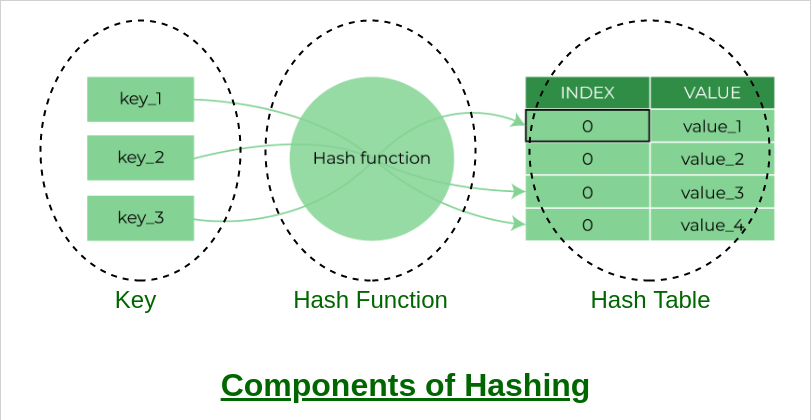Components of hashing

Hash tables are generally implemented using arrays and the performance of hashing data structure depends upon these three factors:

1. Hash Function
2. Size of the Hash Table
3. Collision Handling Method

### Operation on Hash data structure:

• Insert: This operation is used to map the key-value pair and store this mapping record in the hash data structure.
• Search: This operation is used to search the value of the key in the hash table.
• Delete: This operation is used to delete the stored key-value pair from the hash table.

### Advantages of Hash Data structure

• Hash provides better synchronization than other data structures.
• Hash tables are more efficient than search trees or other data structures
• Hash provides constant time for searching, insertion, and deletion operations on average.

### Applications of Hash Data structure:

• Hash is used in databases for indexing.
• Hash is used in disk-based data structures.
• In some programming languages like Python, JavaScript hash is used to implement objects.

## Conclusion

Data structures and algorithms are dependent on each other. We use a well-suited data structure to apply algorithms. Similarly, we apply algorithms to the data structure. And it is also clear from the definition that data structure stores the unstructured data in an organized form whereas algorithms are the set of instructions that a computer follows to solve a particular task.

Data structures are the building blocks of Algorithms, and Algorithms are the platforms upon which Data Structures are applied and tested.

With all the cases put forward, and after discussing the merits and demerits of top data structures that every programmer must know, it is important that you start learning Data Structures first, but do not dig deep into them without the knowledge of Algorithms.

Related articles: